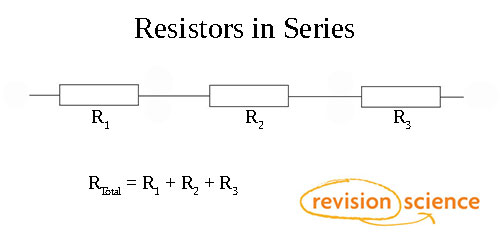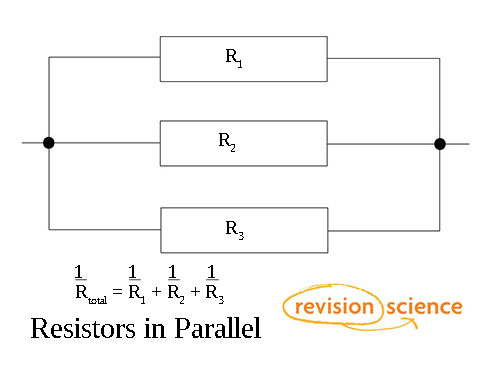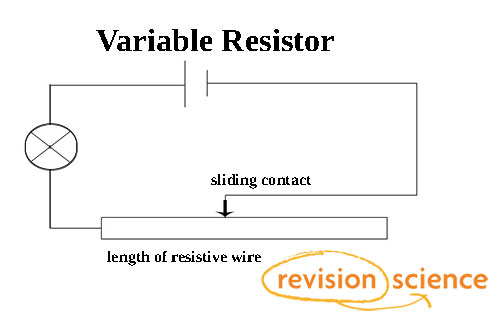## Title

Resistance in Circuits

Series Circuit

RTotal = R1 + R2 + R3 + ... + RnParallel Circuit

1/RTotal = 1/R1 + 1/R2 + 1/R3 + ... + 1/RN

The total resistance is always less than the smallest value of resistance.Internal Resistance

A cell in a circuit has its own internal resistance, r. The greater the cell’s current the more work is done against the cell’s internal resistance, and therefore less can be done on the external circuit.

emf = terminal p.d. + p.d. across internal resistance

E = V + Ir

Variable Resistors

A variable resistor allows for finer control over the amount of resistance.

It could be a mechanism which changes the length of wire through which current must flow, as seen in the example below:Energy Transfer and Power

Resisters heat up as current passes through them. The energy transferred by heating can be found by the following:

ΔE = V I Δt (Energy equals Voltage x Current x Time)

Power is the rate of working or rate of energy transfer.

P = I V (Power = Current x Voltage).

Given the resistance equation we can work out:

P = I2R